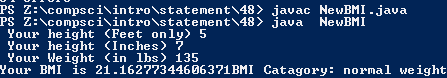# Assignemnt #48

## Code

```///colin hinton
///5th period
///New BMI
///NewBMI.java
///10/19/2015

import java.util.Scanner;

class NewBMI
{
public static void main (String [] args )
{
Scanner keyboard = new Scanner(System.in);
double weight, bmi, meters, metersNew, kg, kgNew;
int inches, feet;

meters = .0254;
kg = .454;
System.out.print(" Your height (Feet only) ");
feet = keyboard.nextInt();

inches = keyboard.nextInt();

metersNew = ((( feet * 12) + inches) * meters);

System.out.print(" Your Weight (in lbs) ");
weight = keyboard.nextDouble();

kgNew = weight * kg;
bmi = kgNew / (metersNew * metersNew);

if (bmi < 15.0)
{
System.out.print("Your BMI is " + bmi);
System.out.print("BMI Catagory: Very severely underweight");
}
else if ((bmi >= 15.0) && (bmi <= 16.0))
{
System.out.print("Your BMI is " + bmi);
System.out.print("BMI Catagory: severely underweight");
}
else if ((bmi >= 16.1) && (bmi <= 18.4))
{
System.out.print("Your BMI is " + bmi);
System.out.print("BMI Catagory: underweight");
}
else if ((bmi >= 18.5) && (bmi <= 24.9))
{
System.out.print("Your BMI is " + bmi);
System.out.print("BMI Catagory: normal weight");
}
else if ((bmi >=25.0) && (bmi <=29.9))
{
System.out.print("Your BMI is " + bmi);
System.out.print("BMI Catagory: overweight");
}
else if ((bmi >= 30.0) && (bmi <= 34.9))
{
System.out.print("Your BMI is " + bmi);
System.out.print("BMI Catagory: moderately obese");
}
else if ((bmi >= 35.0) && (bmi <= 39.9))
{
System.out.print("Your BMI is " + bmi);
System.out.print("BMI Catagory:  severely overweight");
}
else if ( bmi >= 40.0)
{
System.out.print("Your BMI is " + bmi);
System.out.print("BMI Catagory: Very severely overweight");
}
else
{
System.out.print("you done goofed");
}

}
}

```

### Picture of the output